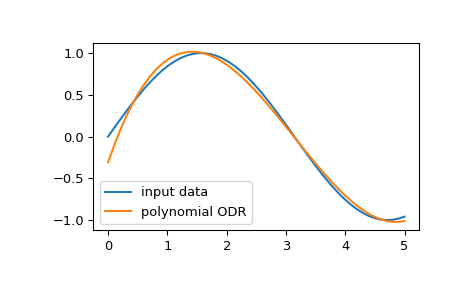# scipy.odr.polynomial¶

scipy.odr.polynomial(order)[source]

Factory function for a general polynomial model.

Parameters
orderint or sequence

If an integer, it becomes the order of the polynomial to fit. If a sequence of numbers, then these are the explicit powers in the polynomial. A constant term (power 0) is always included, so don’t include 0. Thus, polynomial(n) is equivalent to polynomial(range(1, n+1)).

Returns
polynomialModel instance

Model instance.

Examples

We can fit an input data using orthogonal distance regression (ODR) with a polynomial model:

>>> import matplotlib.pyplot as plt
>>> from scipy import odr
>>> x = np.linspace(0.0, 5.0)
>>> y = np.sin(x)
>>> poly_model = odr.polynomial(3)  # using third order polynomial model
>>> data = odr.Data(x, y)
>>> odr_obj = odr.ODR(data, poly_model)
>>> output = odr_obj.run()  # running ODR fitting
>>> poly = np.poly1d(output.beta[::-1])
>>> poly_y = poly(x)
>>> plt.plot(x, y, label="input data")
>>> plt.plot(x, poly_y, label="polynomial ODR")
>>> plt.legend()
>>> plt.show()#### Previous topic

scipy.odr.OdrStop

#### Next topic

scipy.odr.exponential